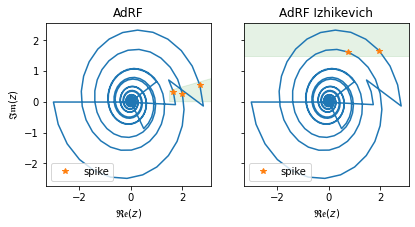# Dynamics, Neurons, and SpikesDynamics are the fundamental building blocks of neurons in lava.dl.lib.slayer. In this tutorial, we will go throught some fundamental neuron dynamics that are built in SLAYER and illustrate how they can be combined to build a variety of neuron models. These dynamics are custom CUDA accelerated, fixed precision compatible, and PyTorch autogard compatible with learnable decay(s) and persistent state(s).

Dynamics scaling: the internal dynamics computation are done in fixed precision range. However, the parameters and state can be interpreted in scaled representation. This has two advantages.

• First, the dynamics are scaled such that the backpropagation gradients are usually in proper range for good gradient flow thus eliminating the need for unnatural scaling of surrogate gradients.

• Second, the states and decays are in intuitive range rather than in abstract scaled fixed point state.

## Notations:

Following notation for variable is used in this notebook.

Notation

Variable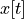inputstate variable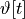threshold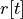refractory state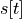spike flag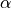leak parameter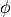phase shift

NOTE: this is a deep dive tutorial. It introduces * neuron dynamics in SLAYER. * how some available neurons are implemented. * how one can use these dynamics to build their custom neuron model. * real and complex spike mechanism in SLAYER.

:

import numpy as np
import matplotlib.pyplot as plt
import torch

import lava.lib.dl.slayer as slayer


# Parameter setup

:

device = torch.device('cpu')
# device = torch.device('cuda')

:

time = np.arange(1000)
t = torch.FloatTensor(time).to(device)
input = torch.zeros_like(t)
for tt, ww in [[10, 1], [97, 1.8], [100, 1.6], [270, -3], [500, 0.5]]:
input[tt] = ww

:

scale = 1<<12 # scale factor for integer simulation
decay = torch.FloatTensor([0.1 * scale]).to(device)
initial_state = torch.FloatTensor().to(device)
threshold = 1.5


# Dynamics

Leaky integrator is the basic first order neuron dynamics represented by the following discrete system:

state dynamics: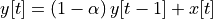spike dynamics: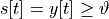reset dynamics: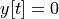Leaky integrator dynamics can be cascaded with other dynamics to form a second order neuron like CUBA neuron and other higer order neurons.

:

y = slayer.neuron.dynamics.leaky_integrator.dynamics(input, decay=decay, state=initial_state, w_scale=scale, threshold=threshold)


Fully backpropagable spike mechanism is avalilable as slayer.spike.Spike. It supports binary as well as graded spikes.

:

sp = slayer.spike.Spike.apply(
y,
threshold,
1, # tau_rho: gradient relaxation constant
1, # scale_rho: gradient scale constant
0, # voltage_last: voltage at t=-1
1, # scale: graded spike scale
)


## 1.1 CUrrent BAsed (CUBA) leaky integrate and fire (LIF) neuron

A CUBA-LIF neuron is simply the leaky integrator dynamics applied to current followed by voltage. For easy usage, CUBA neuron is avaliable as slayer.neuron.cuba.

:

second_order_th = threshold * 5
current = slayer.neuron.dynamics.leaky_integrator.dynamics(input, decay=decay, state=initial_state, w_scale=scale)
voltage = slayer.neuron.dynamics.leaky_integrator.dynamics(current, decay=decay, state=initial_state, w_scale=scale, threshold=second_order_th)


## 1.2 Plot results

:

fig,ax = plt.subplots(3, 1, figsize=(15, 7))
ax.plot(time, input.cpu(), label='weighted spikes')
ax.legend(loc='upper right')

ax.plot(time, y.cpu(), label='Leaky integrator dynamics')
ax.plot(time, threshold * np.ones_like(time), alpha=0.5, label='threshold')
ax.plot(time[sp>0], y[sp>0], '*', label='spike')
ax.legend(loc='upper right')

ax.plot(time, current.cpu(), label='CUBA current')
ax.plot(time, voltage.cpu(), label='CUBA voltage')
ax.plot(time, second_order_th * np.ones_like(time), alpha=0.5, label='threshold')
ax.plot(time[voltage>second_order_th], voltage[voltage>second_order_th].cpu(), label='spike')
ax.legend(loc='upper right')

ax[-1].set_xlabel('time')

:

Text(0.5, 0, 'time')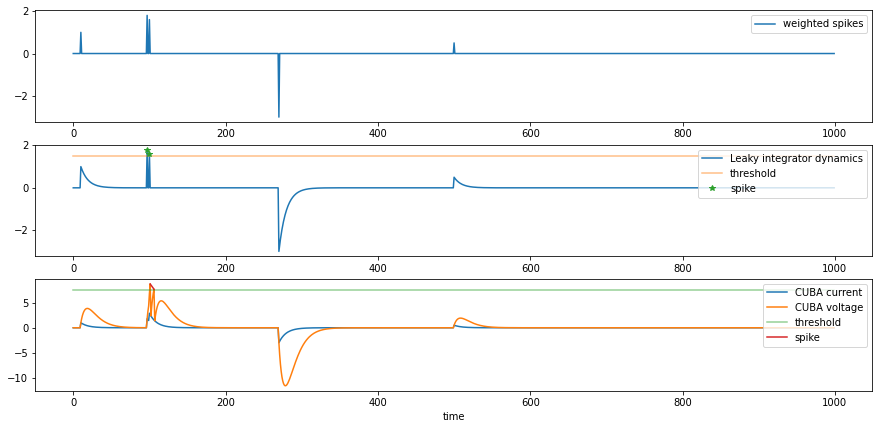threshold dynamics: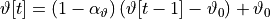refractory dynamics: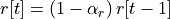spike dynamics: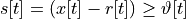post spike dynamics:and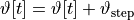## 2.1 Adaptive Leaky Integrator and Fire Neuron

When coupled with a second order leaky integrator, it results in second order adaptive leaky integartor neuron. For easy usage, ALIF neuron is avaliable as slayer.neuron.alif.

:

current = slayer.neuron.dynamics.leaky_integrator.dynamics(input, decay=decay, state=initial_state, w_scale=scale)
voltage = slayer.neuron.dynamics.leaky_integrator.dynamics(current, decay=decay, state=initial_state, w_scale=scale)
voltage,                      # dynamics state
ref_state=initial_state,      # previous refractory state
ref_decay=0.5*decay,          # refractory decay
th_state=initial_state + second_order_th, # previous threshold state
th_decay=decay,               # threshold decay
th_scale=0.5*second_order_th, # threshold step
th0=second_order_th,          # threshold stable state
w_scale=scale                 # fixed precision scaling
)


## 2.2 Plot results

:

fig,ax = plt.subplots(2, 1, figsize=(15, 4.5))
ax.plot(time, input.cpu(), label='weighted spikes')
ax.legend(loc='upper right')

ax.plot(time, current.cpu(), label='ALIF current')
ax.plot(time, voltage.cpu(), label='ALIF voltage')
ax.plot(time, ref.cpu(), label='refractory dynamics')
ax.plot(time, th.cpu(), alpha=0.5, label='threshold')
ax.plot(time[(voltage-ref)>th], voltage[(voltage-ref)>th], '*', label='spike')
ax.legend(loc='upper right')

ax[-1].set_xlabel('time')

:

Text(0.5, 0, 'time')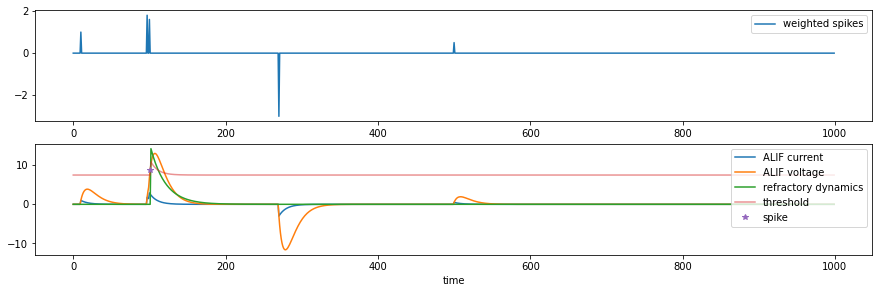### 3. Resonator

Resonator is first order complex leaky dynamics. The leak is, in general, complex and gives rise to oscillatory dynamics. The resonator dynamics is described by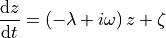where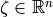is the complex input to the system,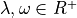.

Discretization

The impulse response of resonator isThe coresponding discrete system has the impulse respnse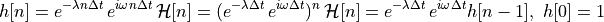The equivalent discrete system is therefore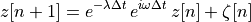The complex decay can be decoupled as

magnitude leak: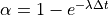phase shift:decay matrix: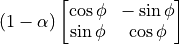## 3.1 Resonate and Fire (RF) neuron

A resonate and fire neuron spikes when it’s internal state crosses the real axis in the positive real half plane greater than the neuron’s theshold,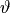. Formally, it can be stated as follows.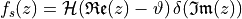or equivalently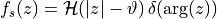For easy usage, RF neuron is avaliable as slayer.neuron.rf.

:

re_input = input
im_input = 2*torch.randn_like(re_input) * (re_input > 0)
alpha = torch.FloatTensor([0.03 * scale]).to(device)
phi = 2 * np.pi /25
sin_decay = (scale-alpha) * np.sin(phi)
cos_decay = (scale-alpha) * np.cos(phi)
re, im = slayer.neuron.dynamics.resonator.dynamics(
re_input, im_input,
sin_decay, cos_decay,
real_state=initial_state,
imag_state=initial_state,
w_scale=scale,
)



Fully backpropagable complex spike mechanism supporting phase spiking mechanism of RF neuron is avalilable as slayer.spike.complex.Spike. It supports binary as well as graded spikes.

spike dynamics: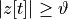and:

sp = slayer.spike.complex.Spike.apply(
re, im,
threshold,
1, # tau_rho: gradient relaxation constant
1, # scale_rho: gradient scale constant
0, # voltage_last: voltage at t=-1
1, # scale: graded spike scale
)


## 3.2 Izhikevich Resonate and Fire (RF-Iz) neuron

RF-Izhikevich neuron dynamics is same as the basic RF neuron. However the firing and reset mechanism is different. The neuron fires when the imaginary state is above threshold and the real state is reset to zero post spike.

spike dynamics: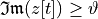post spike dynamics: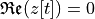For easy usage, RF-Izhikevich neuron is avaliable as slayer.neuron.rf_iz.

:

iz_re, iz_im = slayer.neuron.dynamics.resonator.dynamics(
re_input, im_input,
sin_decay, cos_decay,
real_state=initial_state,
imag_state=initial_state,
w_scale=scale,
threshold=threshold,
)


## 3.3 Second Order RF neuron

Two resonator dynamics can be cascaded to produce Gammatone like second order resonator dynamics. In theory, it could also be combined with leaky integrator for even more exotic neuron model.

:

second_order_th = threshold * 15
re_0, im_0 = slayer.neuron.dynamics.resonator.dynamics(
re_input, im_input,
sin_decay, cos_decay,
real_state=initial_state,
imag_state=initial_state,
w_scale=scale,
)
re_1, im_1 = slayer.neuron.dynamics.resonator.dynamics(
re_0, im_0,
sin_decay, cos_decay,
real_state=initial_state,
imag_state=initial_state,
w_scale=scale,
)
sp_1 = slayer.spike.complex.Spike.apply(re_1, im_1, second_order_th, 1, 1, False, 0, 1)


## 3.4 Plot results

:

fig,ax = plt.subplots(4, 1, figsize=(15, 9))
ax.plot(time, re_input.cpu(), label='real weighted spikes')
ax.plot(time, im_input.cpu(), label='imag weighted spikes')
ax.legend(loc='upper right')

ax.plot(time, re.cpu(), label='RF real state')
ax.plot(time, im.cpu(), label='RF imag state')
ax.plot(time, threshold * np.ones_like(time), alpha=0.5, label='threshold')
ax.plot(time[sp>0], re[sp>0], '*', label='spike')
ax.legend(loc='upper right')

ax.plot(time, iz_re.cpu(), label='RF Izhikevich real state')
ax.plot(time, iz_im.cpu(), label='RF Izhikevich imag state')
ax.plot(time, threshold * np.ones_like(time), alpha=0.5, label='threshold')
ax.plot(time[iz_im > threshold], iz_im[iz_im > threshold], '*', label='spike')
ax.legend(loc='upper right')

ax.plot(time, re_1.cpu(), label='RF-Or2 real state')
ax.plot(time, im_1.cpu(), label='RF-Or2 imag state')
ax.plot(time, second_order_th * np.ones_like(time), alpha=0.5, label='threshold')
ax.plot(time[sp_1>0], re_1[sp_1>0], '*', label='spike')
ax.legend(loc='upper right')

ax[-1].set_xlabel('time')

:

Text(0.5, 0, 'time')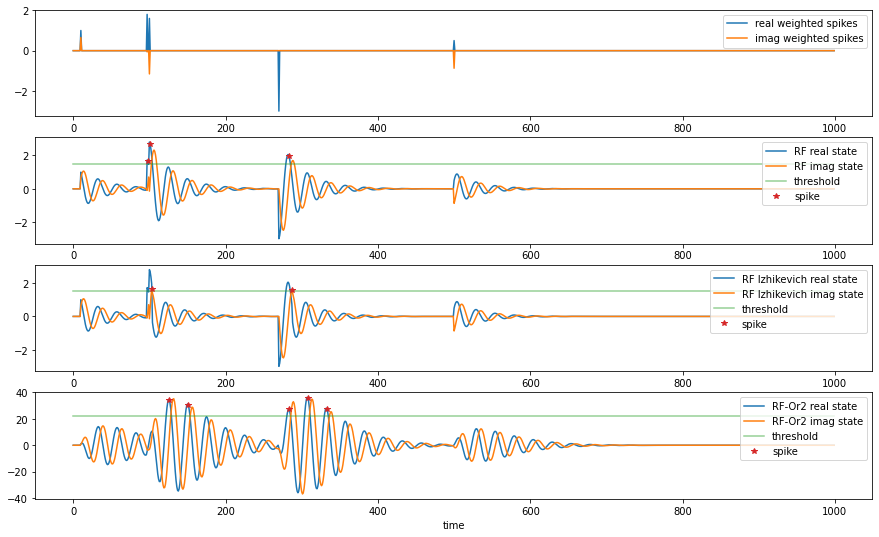:

def plot_phase_region(ax, threshold, sin_decay):
xlims = ax.get_xlim()
ylims = ax.get_ylim()
xx = np.array([threshold, threshold+500])
yy = xx * sin_decay
ax.fill_between(xx, yy, color='green', alpha=0.1)
ax.set_xlim(xlims)
ax.set_ylim(ylims)

def plot_iz_region(ax, threshold):
ylims = ax.get_ylim()
ax.axhspan(threshold, threshold+50, color='green', alpha=0.1)
ax.set_ylim(ylims)

:

fig, ax = plt.subplots(1, 3, figsize=(10, 3))
ax.plot(re.cpu(), im.cpu())
ax.plot(re[sp>0], im[sp>0], '*', label='spike')
plot_phase_region(ax, threshold, sin_decay.item()/scale)
ax.set_xlabel('$\mathfrak{Re}(z)$')
ax.set_ylabel('$\mathfrak{Im}(z)$')
ax.legend(loc='lower left')
ax.set_title('RF')
ax.plot(iz_re.cpu(), iz_im.cpu())
ax.plot(iz_re[iz_im > threshold], iz_im[iz_im > threshold], '*', label='spike')
plot_iz_region(ax, threshold)
ax.set_xlabel('$\mathfrak{Re}(z)$')
ax.legend(loc='lower left')
ax.set_title('RF Izhikevich')

ax.plot(re_1.cpu(), im_1.cpu())
ax.plot(re_1[sp_1>0], im_1[sp_1>0], '*', label='spike')
plot_phase_region(ax, second_order_th, sin_decay.item()/scale)
ax.set_xlabel('$\mathfrak{Re}(z)$')
ax.legend(loc='lower left')
ax.set_title('RF-Or2')

:

Text(0.5, 1.0, 'RF-Or2')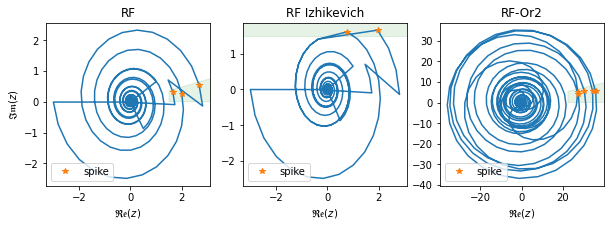Adaptive resonator adds adaptive threshold and refractory dynamics on top of resonator. Two flavors of adaptive resonator dynamics are available in SLAYER: slayer.neuron.dynamics.phase_th and slayer.neuron.dynamics.adaptive_resonator corresponding to phase spiking and Izhikevich spiking mechanisms respectively. Both dynamics follow the same post spike dynamics.

post spike dynamics:andAdRF neuron spikes when its state crosses zero phase with real value higher than refractory dynamics and threshold dynamics combined.

spike dynamics: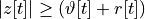andFor easy usage, AdRF neuron is avaliable as slayer.neuron.adrf.

:

adrf_re, adrf_im = slayer.neuron.dynamics.resonator.dynamics(
re_input, im_input,
sin_decay, cos_decay,
real_state=initial_state,
imag_state=initial_state,
w_scale=scale,
)
im_state=initial_state, # only imaginary state is needed to determine first phase crossing
ref_state=initial_state, ref_decay=0.5*decay,       # refractory state and decay
th_state=initial_state + threshold, th_decay=decay, # threshold state and decay
th_scale=0.5 * threshold, # threshold step
th0=threshold,          # threshold stable state
w_scale=scale,
)
1, # tau_rho: gradient relaxation constant
1, # scale_rho: gradient scale constant
0, # voltage_last: voltage at t=-1
1, # scale: graded spike scale
)


AdRF-Iz neuron fires when the imaginary state exceeds the threshold and refractory dynamics. There is no hard reset in this model.

spike dynamics: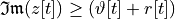For easy usage, AdRF-Iz neuron is avaliable as slayer.neuron.adrf_iz.

:

adrf_iz_re, adrf_iz_im, adrf_iz_th, adrf_iz_ref = slayer.neuron.dynamics.adaptive_resonator.dynamics(
re_input, im_input,
sin_decay, cos_decay, ref_decay=0.5*decay, th_decay=decay,
real_state=initial_state,
imag_state=initial_state,
ref_state=initial_state,
th_state=initial_state + threshold,
th_scale=0.5 * threshold, # threshold step
th0=threshold,          # threshold stable state
w_scale=scale,
)



## 4.3 Plot results

:

fig,ax = plt.subplots(3, 1, figsize=(15, 6.6))
ax.plot(time, re_input.cpu(), label='real weighted spikes')
ax.plot(time, im_input.cpu(), label='imag weighted spikes')
ax.legend(loc='upper right')

ax.legend(loc='upper right')

ax.legend(loc='upper right')

ax[-1].set_xlabel('time')

:

Text(0.5, 0, 'time')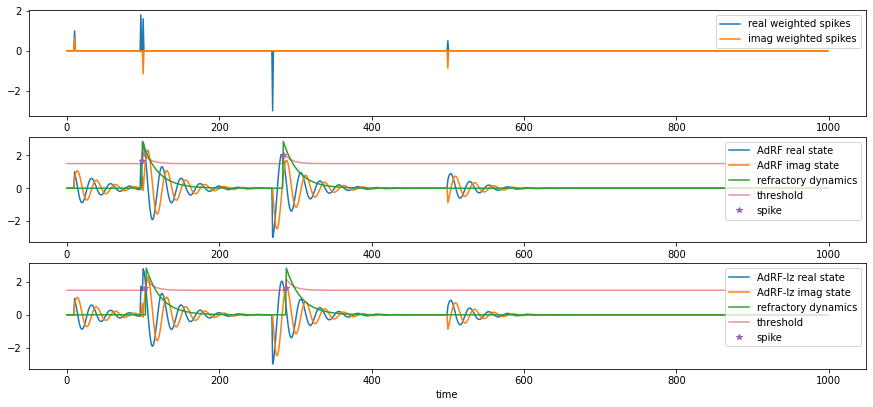:

import matplotlib.patches as patches

fig, ax = plt.subplots(1, 2, figsize=(6.5, 3), sharey=True)
plot_phase_region(ax, threshold, sin_decay.item()/scale)
ax.set_xlabel('$\mathfrak{Re}(z)$')
ax.set_ylabel('$\mathfrak{Im}(z)$')
ax.legend(loc='lower left')
ax.set_xlabel('$\mathfrak{Re}(z)$')

:

Text(0.5, 1.0, 'AdRF Izhikevich')